### 4. MAXIMUM-LIKELIHOOD METHOD

The preceding section was devoted to the case in which one had a discrete set of hypotheses among which to choose. It is more common in physics to have an infinite set of hypotheses; i.e., a parameter that is a continuous variable. For example, in the µ-e decay distribution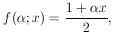the possible values for0 belong to a continuous rather than a discrete set. In this case, as before, we invoke the same basic principle which says the relative probability of any two different values ofis the ratio of the probabilities of getting our particular experimental results, xi, assuming first one and then the other, value ofis true. This probability function ofis called the likelihood function,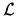().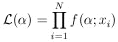(2) The likelihood function,(), is the joint probability density of getting a particular experimental result, x1, ... , xn, assuming f (;x) is the true normalized distribution function: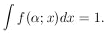The relative probabilities ofcan be displayed as a plot of() vs.. The most probable value ofis called the maximum-likelihood solution*. The rms (root-mean-square) spread ofabout* is a conventional measure of the accuracy of the determination=* . We shall call this.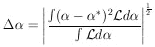(3)

In general, the likelihood function will be close to Gaussian (it can be shown to approach a Gaussian distribution as N ->) and will look similar to Fig. 1b.

Fig. 1a represents what is called a case of poor statistics. In such a case, it is better to present a plot of() rather than merely quoting* and. Straightforward procedures for obtainingare presented in Sections 6 and 7.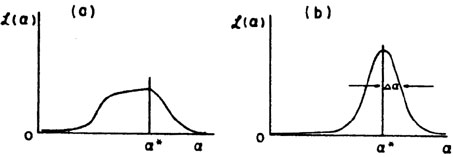Figure 1. Two examples of likelihood functions().

A confirmation of this inverse probability approach is the Maximum-Likelihood Theorem, which is proved in Cramer  by use of direct probability. The theorem states that in the limit of large N,* ->0; and furthermore, there is no other method of estimation that is more accurate.

In the general case in which there are M parameters,1, ...,M, to be determined, the procedure for obtaining the maximum likelihood solution is to solve the M simultaneous equations,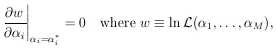(4)